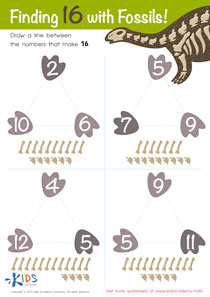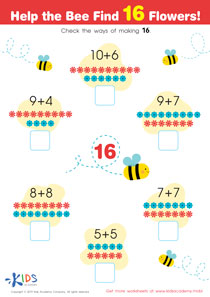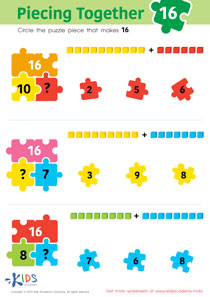# Math Lesson - Making 16, Kindergarten

• ### Activity 1 / Finding 16 With Fossils WorksheetFossils are bones of things that have been long dead. Experts hunt for these fossils, dig them up, study them, and then learn some more about that particular thing such as how it lived, what it looked like, and when it lived. Show your kids the picture in this worksheet and point out the fossils there.  In each triangle, there are 3 numbers; two of these numbers when added make 16. Then, help them count the bones and draw a line between the numbers that make 16.

• ### Activity 2 / Help the Bee Find 16 Flowers WorksheetBefore beginning this worksheet, you might want to refresh your students’ mind a little bit by asking them to count as high as they can. Now, teach them that different numbers added together can give the same figure. Give your kids simple equations, like adding 2+2 =4, and also 3+1 =4. Ask your students if they can give you other examples, and then look at the simple additions in this printout. Help the bees find 16 flowers by checking the ways of making 16.

• ### Activity 3 / Piecing Together 16 WorksheetUsing puzzles and bright colors to teach your preschoolers is one of the surest and best ways they can learn. Combine learning with some fun with this colorful worksheet. Look at the picture here with your students, and ask them what they see. There are simple mathematical equations on the puzzle pieces, and one equation is missing on each. Help your kids solve the problems, and then circle the puzzle piece that will make the bottom puzzle piece numbers equal 16.

?